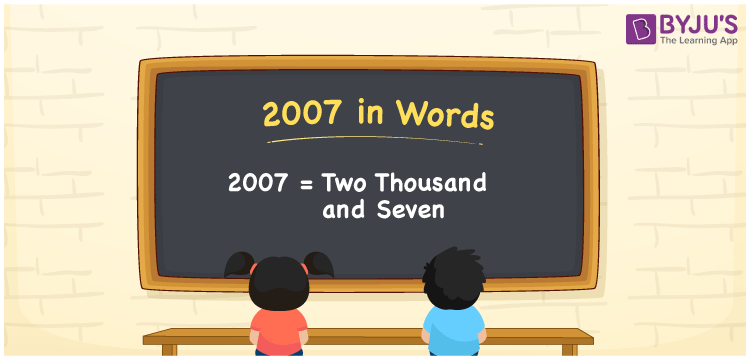# 2007 in Words

We can write 2007 in words as Two thousand and seven. Suppose you bought a movable table that costs Rs. 2007, then you can say, “I bought a movable table worth Two thousand and seven rupees”. Here, the word form “Two thousand and seven” is called the number name of 2007. Let’s learn how to derive the cardinal number 2007 into words here in this article, along with some interesting facts about 2007.

 2007 in words Two thousand and seven Two thousand and seven in Numbers 2007

## 2007 in English Words

We generally express numbers in words using the English alphabet. So, we can write 2007 in English as “Two thousand and seven”.## How to Write 2007 in Words?

The below table is called the place value chart for the number 2007. This chart shows the place values for all the digits of the number 2007.

 Thousands Hundreds Tens Ones 2 0 0 7

Here, ones = 7, tens = 0, hundreds = 0, thousands = 2

Now, by expanding these digits, we get;

2 × Thousand + 0 × Hundred + 0 × Ten + 7 × One

= 2 × 1000 + 0 × 100 + 0 × 10 + 7 × 1

= 2000 + 7

= Two thousand + Seven

= Two thousand and seven

Thus, 2007 in words = Two thousand and seven.

As we know, 2007 is a natural number that precedes 2008 and succeeds 2006.

2007 in words – Two thousand and seven

Is 2007 an odd number? – Yes

Is 2007 an even number? – No

Is 2007 a prime number? – No

Is 2007 a composite number? – Yes

Is 2007 a perfect square number? – No

Is 2007 a perfect cube number? – No

## Frequently Asked Questions on 2007 in Words

Q1

### How I can write 2007 in words?

You can write 2007 in words as “Two thousand and seven”.
Q2

### How do you say 2007 in English words?

You can spell the number 2007 in English words as Two thousand and seven.
Q3

### Express the date 11-oct-2007 in words.

We can express the date 11-oct-2007 in words as “Eleventh October Two thousand and seven”.